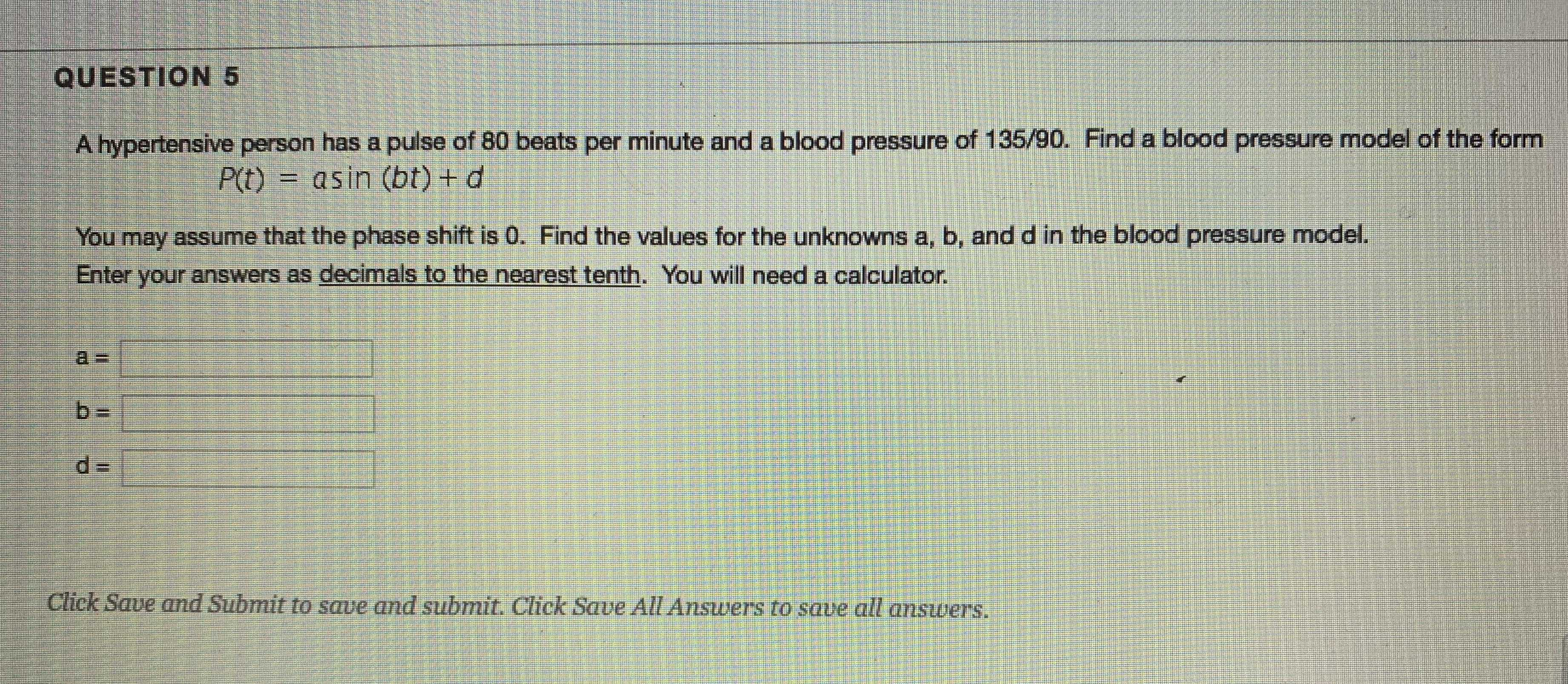### ¿Todavía tienes preguntas de matemáticas?

Pregunte a nuestros tutores expertos
Algebra
PreguntaA hypertensive person has a pulse of $$80$$ beats per minute and a blood pressure of $$135 / 90$$ . Find a blood pressure model of the form

$$P ( t ) = a \sin ( b t ) + d$$

You may assume that the phase shift is 0. Find the values for the unknowns a, b, and d in the blood pressure model. Enter your answers as decimals to the nearest tenth. You will need a calculator.

$$a =$$

$$b =$$

$$d =$$